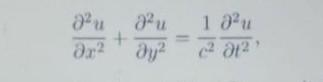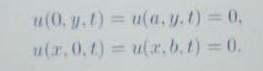# Question Solve the wave equation by the method of separation of variables. The boundary conditions for this system that describes the vibration of a rectangular membrane of area a · b is that at the edges the membrane cannot vibrate, that is: Find the natural frequencies of this membrane and show that there is a degeneracy in the natural frequencies. 1 24 au Or2 ду? + u(0.3.1) = u(ay. 1) = 0, (2.0.1) = urb.1) =0.Solve the wave equationby the method of separation of variables. The boundary conditions for this system that describes the vibration of a rectangular membrane of area a · b is that at the edges the membrane cannot vibrate, that is:Find the natural frequencies of this membrane and show that there is a degeneracy in the natural frequencies.

Transcribed Image Text: 1 24 au Or2 ду? + u(0.3.1) = u(ay. 1) = 0, (2.0.1) = urb.1) =0.
More
Transcribed Image Text: 1 24 au Or2 ду? + u(0.3.1) = u(ay. 1) = 0, (2.0.1) = urb.1) =0.